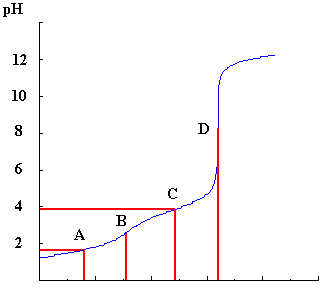# Titrations Help File

### Calculations

Here is an example of a titration curve for a difunctional acid (H2A) titrated against KOH.From the lines from positions A,B,C and D, the concentration of the acid and its pK values can be determined.

### Determination of Concentration

The reactions which occur during the titration are:

```				H2A	->	HA-	+	H+

HA-	->	A2-	+	H+
```

Point B, represents the position of the end-point of the reaction between H2A and HA-, that is, when one mole of protons has been removed from H2A by reaction with one mole of base and the point where HA- now fully exists.

If you used the default concentration of KOH (0.100 mol dm-3) then the number of moles of base is:

```						0.100 x B / 1000
= B x 10-4
```

If we let the concentration of the acid be represented by X and given that the volume of acid added to the beaker is 16 cm3, then the number of moles of acid is given by:

```						X x 16 / 1000
```

Since at point B the number of moles of base is equal to the number of moles of acid, these two expressions are equivalent and by reading off the volume at B from the curve it is possible to calculate X.

For example, if B=16 cm3, then X=0.100 mol dm-3.

Point D represents the position of the end-point of the reaction between HA- and A2-. This must be at twice the volume at B, since both protons have now been removed and each would require the same amount of base to neutralise them.

### Determination of pK values

The calculations needed to determine the pK values can best be seen by writing the equilibrium expressions for the reactions above in terms of pK and pH.

For the first step:

```				Ka = [H+].[HA-]/ [H2A]

or		[H2A]/[HA-] = [H+]/Ka

hence		log10 [H2A]/[HA-] = pKa - pH
```

When the concentrations of H2A and HA- are equal, then the left hand side of this expression becomes zero (log 1=0.0) and at that point the pKa1 is equal to the pH.

From the titration curve, this is represented by point A where the volume is half that of B. At this point, half of the original H2A is converted to HA- and there is a 50:50 mixture of both species present in the solution.

Similarly the point C, which is 1.5 times the volume at point B and 3 times the volume at A, can be used to determine pKa2 since at this point there is a 50:50 mixture of the species HA- and A2- and so again the pK can be read off the pH axis.

In the example given, pK1 is =1.6 and pK2 =3.9.

Further information on pH and logarithms is available.Return to Chemistry, UWI-Mona, Home Page

May-96, rjl